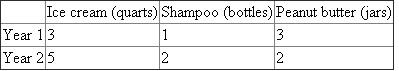# Quiz 24: An Introduction to Macroeconomics

We can first put the information we have been given into a table:We are given that the prices in both years of ice cream, shampoo, and peanut butter are \$4, \$3, and \$2, respectively. Remember that the nominal GDP of an economy in any given year is simply the value of all the things that were produced in that year. Thus, we see that we can find year 1's GDP by adding the value of the ice cream produced, the value of the shampoo produced, and the value of the peanut butter produced together. In other words:We can use the same method to find the nominal GDP in year 2. Note that while the prices did not change, but the quantity produced in year two did change. So, we have:Here, it is easiest to use the expenditures approach for calculating GDP, since we are already given all the components. Remember that the expenditures approach says that the GDP of a country is simply the sum of the consumption, investment, net exports, and government spending in any given year. First, we see that there are two types of consumption that we have been given. The first is personal consumption of \$50 billion and the second is purchases of secondhand items of \$8 billion. Note that when we look at consumption, we only look at newly produced final goods, so purchases of secondhand item actually does not count towards the GDP in that year. Secondly, we are given that purchases of stocks and bonds are \$30 billion and gross investment is \$25 billion. Purchases of stocks and bonds are referred to as noninvestment transactions, so they do not count towards the investment portion of GDP. Thus, we only count the \$25 billion of gross investment as part of the investment portion of GDP. Thus, we have: